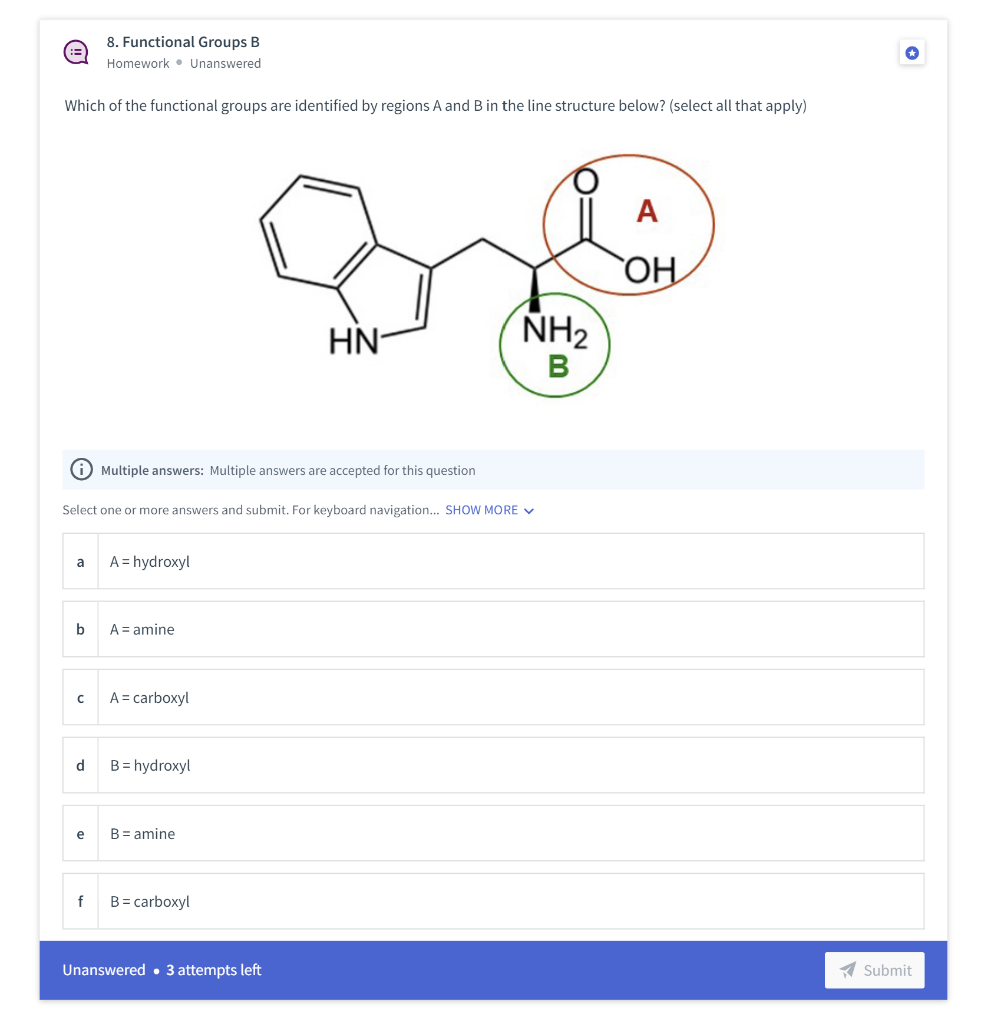# Question Which of the functional groups are identified by regions A and B in the line structure below? (select all that apply) Which of the functional groups are identified by regions $$A$$ and $$B$$ in the line structure below? (select all that apply) Multiple answers: Multiple answers are accepted for this question Select one or more answers and submit. For keyboard navigation... SHOW MORE $$\checkmark$$ a $$\quad A=$$ hydroxyl b $$\quad A=$$ amine c $$\quad \mathrm{A}=$$ carboxyl d $$\quad B=$$ hydroxyl e $$\quad B=$$ amine Unanswered $$\cdot 3$$ attempts leftTranscribed Image Text: Which of the functional groups are identified by regions $$A$$ and $$B$$ in the line structure below? (select all that apply) Multiple answers: Multiple answers are accepted for this question Select one or more answers and submit. For keyboard navigation... SHOW MORE $$\checkmark$$ a $$\quad A=$$ hydroxyl b $$\quad A=$$ amine c $$\quad \mathrm{A}=$$ carboxyl d $$\quad B=$$ hydroxyl e $$\quad B=$$ amine Unanswered $$\cdot 3$$ attempts left
Transcribed Image Text: Which of the functional groups are identified by regions $$A$$ and $$B$$ in the line structure below? (select all that apply) Multiple answers: Multiple answers are accepted for this question Select one or more answers and submit. For keyboard navigation... SHOW MORE $$\checkmark$$ a $$\quad A=$$ hydroxyl b $$\quad A=$$ amine c $$\quad \mathrm{A}=$$ carboxyl d $$\quad B=$$ hydroxyl e $$\quad B=$$ amine Unanswered $$\cdot 3$$ attempts left